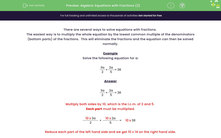# Solve Equations with Fractions

In this worksheet, students will solve equations with fractions.Key stage:  KS 3

Curriculum topic:   Algebra

Curriculum subtopic:   Understand Expressions, Equations, Inequalities, Terms and Factors

Popular topics:   Algebra worksheets

Difficulty level:#### Worksheet Overview

There are several ways to solve equations with fractions.

The easiest way is to multiply the whole equation by the lowest common multiple of the denominators (bottom parts) of the fractions.  This will eliminate the fractions and the equation can then be solved normally.

Example

Solve the following equation for a:

 3a + 2a = 38 2 5

 3a + 2a = 38 2 5

Multiply both sides by 10, which is the LCM of 2 and 5.

Each part must be multiplied.

 10 x 3a + 10 x 2a = 10 x 38 2 5

Simplify each part of the left-hand side and we get 10 x 38 on the right-hand side.

 5 10 x 3a + 2 10 x 2a = 10 x 38 2 5

Simplify:

5 x 3a + 2 x 2a = 380

Simplify again:

15a + 4a = 380

Simplify again:

19a = 380

Divide both sides by 19:

19a ÷ 19 = 380 ÷ 19

Simplify:

a = 20

There's a lot to do with these equations but the secret is to work through it one step at a time, and make sure that you write it down!!

Don't worry if you think this is a lot to remember - you can look back at this page at any point during the activity by clicking on the red button on the right of the screen.

Let's get started.

### What is EdPlace?

We're your National Curriculum aligned online education content provider helping each child succeed in English, maths and science from year 1 to GCSE. With an EdPlace account you’ll be able to track and measure progress, helping each child achieve their best. We build confidence and attainment by personalising each child’s learning at a level that suits them.

Get started